Courses

# Previous Year Questions - Probability Class 10 Notes | EduRev

## Class 10 : Previous Year Questions - Probability Class 10 Notes | EduRev

The document Previous Year Questions - Probability Class 10 Notes | EduRev is a part of the Class 10 Course Mathematics (Maths) Class 10.
All you need of Class 10 at this link: Class 10

Short Answer Type Questions

Q.1. The probability of selecting a blue marble at random from a jar that contains only blue, black and green marbles is 1/5. The probability of selecting a black marble at random from the same jar is 1/4. If the jar contains II green marbles, find the total number of marbles in the jar.    [CBSE, Allahabad 2019]
Ans.
Let the total number of marbles in the jar = x
Probability of selecting a blue marble + Probability of selecting a black marble + Probability of selecting a green marble = 1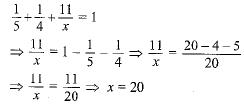Q.2. A die is thrown once. Find the probability of getting a number which
(i) is a prime number
(ii) lies between 2 and 6.    [Delhi 2019]
Ans.
For a die, number of possible outcomes = 6
Prime numbers are 2, 3 and 5.
(i) Probability of getting a prime number = 3/6 = 1/2.
(ii) Probability of getting a number lies between 2 and 6.
= 3/6 = 1/2.

Q.3. A child has a die whose six faces show the letters as given below
A  B  C  A  A  B

The die is thrown once. What is the probability of getting (i) A ? (ii) B?    [CBSE 2019 (30/5/1)]
Ans. The total number of elementary events associated with random experiment of throwing a die is 6.
(i) Let E be the event of getting a letter A .
∴ Favourable number of elementary events = 3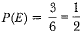(ii) Let E be the event of getting a letter B.
∴ Favourable number of elementary events = 2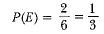Q.4. Two different dice are tossed together. Find the probability:
(i) of getting a doublet
(ii) of getting a sum 10, of the numbers on the two dice.    [CBSE 2018]
Ans.
Possible outcomes are
{(1, 1), (1,2), (1,3), (1,4), (1,5), (1,6)
(2, 1), (2, 2), (2, 3), (2, 4), (2, 5), (2, 6)
(3, 1), (3, 2), (3, 3), (3, 4), (3, 5), (3, 6)
(4, 1), (4, 2), (4, 3), (4, 4), (4, 5), (4, 6)
(5, 1), (5, 2), (5, 3), (5, 4), (5, 5), (5, 6)
(6, 1), (6, 2), (6, 3), (6, 4), (6, 5), (6, 6)}
Total elementary events = 36
(i) Outcomes of doublet = {(1, 1), (2, 2), (3, 3), (4, 4), (5, 5), (6, 6)}, i.e. 6
P(getting a doublet) = 6/36 = 1/6
(ii) Outcomes of getting a sum 10 = {(4, 6), (5, 5), (6, 4)}, i.e. 3
P (getting a sum 10) = 3/36 =1/12.

Q.5. An integer is chosen at random between 1 and 100.
Find the probability that it is:
(i) divisible by 8.
(ii) not divisible by 8.    [CBSE 2018]
Ans.
(i) The integers divisible by 8 between 1 and 100 are 8, 16, 24, 32, 40, 48, 56, 64, 72, 80, 88, 96, i.e. 12
Total outcomes = 98
P(divisible by 8) =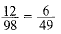(ii) P (not divisible by 8)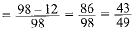Q.6. The probability of selecting a rotten apple randomly from a heap of 900 apples is 0.18. What is the number of rotten apples in the heap?    [AI 2017]
Ans.
Total apples in the heap = 900
∴ Total number of elementary events = 900
One rotten apple is randomly selected from this heap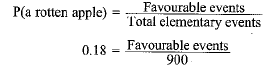⇒ Favourable events = 0.18 x 900 = 162
Hence, there are 162 rotten apples in the heap.

Q.7. If three different coins are tossed together, then find the probability of getting two heads.    [AI 2017 (C)]
Ans.
Three coins are tossed together.
Possible outcomes
{HHH, HHT, HTH, THH, TTT, TTH, THT, HTT}
Number of 2 heads together = 3
Probability of getting two head = 3/8

Q.8. A game consists of tossing a one-rupee coin 3 times and noting the outcome each time. Ramesh wins the game if all the tosses give the same result (i.e. three heads or three tails) and loses otherwise. Find the probability of Ramesh losing the game.     [CBSE (F) 2016, Delhi 2017 (C)]
Ans. The outcomes associated with this experiment are given by HHH, HHT, HTH, THH, TTH, THT, HTT, TTT.
∴ Total number of possible outcomes = 8
Now, Ramesh will lose the game if he gets HHT, HTH, THH, TTH, THT, HTT.
∴ Favourable number of events = 6
∴ Probability that he lose the game = 6/8 = 3/4

Q.9. Two different dice are thrown together. Find the probability that the numbers obtained
(i) have a sum less than 7
(ii) have a product less than 16
(iii) is a doublet of odd number.    [Delhi 2017]
Ans.
Total events associated with the random experiment of throwing two dice are:
(1, 1), (1,2), (1,3), (1,4), (1, 5), (1,6)
(2, 1), (2, 2), (2, 3), (2, 4), (2, 5), (2, 6)
(3, 1), (3,2), (3,3), (3, 4), (3, 5), (3,6)
(4, 1), (4, 2), (4, 3), (4, 4), (4, 5), (4, 6)
(5, 1), (5, 2), (5, 3), (5, 4), (5, 5), (5, 6)
(6, 1), (6, 2), (6, 3), (6, 4), (6, 5), (6, 6)
Total number of elementary events = 6 x 6 = 36
(i) Let A be the event of getting a sum less than 7 in the numbers obtained on the two dice.
∴ Elementary events favourable to event A are:
(1,1), (2,1), (3,1), (4,1), (5,1), (1,2), (1,3), (1,4), (1,5), (2,2), (2,3), (2,4), (3,2), (3,3), (4,2)
Favourable number of elementary events = 15
Hence, required probability = 15/36 = 5/12
(ii) Let A be the event of getting a product less than 16
∴ Elementary events favourable to event A are
(1,1), (1,2), (1,3), (1,4), (1,5), (1,6), (2,1), (2,2), (2,3), (2,4), (2,5), (2,6), (3,1), (3,2), (3,3), (3,4), (3,5), (4,1), (4,2), (4,3), (5,1), (5,2), (5,3), (6,1), (6,2)
⇒ Favourable number of elementary events = 25
Hence, required probability = 25/36
(iii) Let A be the event of doublet of odd numbers. Elementary events favourable to the event A are (1,1), (3,3), (5,5)
∴ Favourable number of elementary events = 3
∴ Required probability = 3/36 = 1/12.

Q.10. A number is chosen at random from the numbers -3,-2,-1,0,1,2,3. What will be the probability that square of this number is less than or equal to 1 ?    [CBSE Delhi 2017]
Ans.
Favourable outcomes are -1, 0, 1 = 3
Total outcomes = 7
∴ Required probability = 3/7

Q.11. Cards marked with number 3, 4, 5 , .... , 50 are placed in a box and mixed thoroughly, A card is drawn at random from the box. Find the probability that the selected card bears a perfect square number.    [CBSE Delhi 2016]
Ans.
Possible outcomes are 4, 9, 16, 25, 36, 49, i.e., 6.
∴ P (perfect square number) = 6/48 or 1/8

Q.12. A card is drawn at random from a well shuffled pack of 52 playing cards. Find the probability o f getting neither a red card nor a queen.    [CBSE-(AI) 2016]
Ans.
Number of possible outcomes = 52
Number of red cards and queens = 28
Number of favourable outcomes = 52 - 28 = 24
P (getting neither a red card nor a queen) = 24/52 = 6/13

Q.13. 20 tickets, on which numbers 1 to 20 are written, are mixed throughly and then a ticket is drawn at random out of them. Find the probability that the number on the drawn ticket is a multiple of 3 or 7.    [CBSE (F) 2016]
Ans.
n(S) = 20, multiples of 3 or 7, A: {3 , 6, 9, 12, 15, 18, 7, 14}, n(A) = 8
∴ Required probability = 8/20 or 2/5

Q.14. Three unbiased coins are tossed together. Find the probability of getting:    [CBSE (AI) 2016]
(i) all heads.
(ii) exactly two heads.
(iii) exactly one head.
(iv) at least two heads.
(v) at least two tails.
Ans.
Elementary events associated to random experiment of tossing three coins are HHH, HHT, HTH, THH, HTT, THT, TTH, TTT
∴ Total number of elementary events = 8
(i) The event “getting all heads” is said to occur, if the elementary event HHH occurs, i.e. favourable number of elementary events = 1
Hence, required probability = 1/8
(ii) The event “getting two heads” will occur, if one of the elementary events HHT, THH, HTH occurs i.e.; favourable number of elementary events = 3
Hence, required probability =3/8
(iii) The event of “getting one head”, when three coins are tossed together, occurs if one of the elementary events HTT, THT, TTH, occurs i.e. favourable number of elementary events = 3
Hence, required probability = 3/8
(iv) If any of the elementary events HHH, HHT, HTH, and THH is an outcome, then we say that the event “getting at least two heads” occurs i.e.; favourable number of elementary events = 4
Hence, required probability = 4/8 = 1/2.
(v) Similar as
(iv) P (getting at least two tails) = 4/8 = 1/2

Q.15. In a single throw of a pair of different dice, what is the probability of getting (i) a prime number on each dice ? (ii) a total of 9 or 11 ?     [CBSE Delhi 2016]
Ans.
Total outcomes = 36
(i) Favourable outcomes are (2,2) (2,3) (2,5) (3,2) (3,3) (3,5) (5,2) (5,3) (5,5) i.e., 9.
P (a prime number on each die) = 9/36 or 1/4
(ii) Favourable outcomes are (3,6) (4,5) (5,4) (6,3) (5,6) (6,5) i.e., 6 outcomes
P (a total of 9 or 11) = 6/36 or 1/6

Long Answer Type Questions

Q.1. A box contains cards numbered from 1 to 20. A card is drawn at random from the box. Find the probability that number on the drawn card is
(i) a prime number
(ii) a composite number
(iii) a number divisible by 3    [CBSE 2018(C)]
Ans.
(i) Prime numbers from 1 to 20 are 2, 3, 5, 7, 11, 13, 17, 19
Number of prime numbers (favourable cases) = 8
Total possible outcomes = 20
P(prime number) = 8/20 = 2/5
(ii) Composite numbers from 1 to 20 are 4, 6, 8, 9, 10, 12, 14, 15, 16, 18, 20
Number of composite numbers (Favourable outcomes) = 11
Total possible outcomes = 20
P(composite numbers) =11/20
(iii) Numbers divisible by 3 are 3,6,9,12,15 and 18
Number of favourable outcomes = 6
Total possible outcomes = 20
P(number divisible by 3) = 6/30 = 3/10

Q.2. The King, Queen and Jack of clubs are removed from a pack of 52 cards and then the remaining cards are well shuffled. A card is selected from the remaining cards. Find the probability of getting a card
(i) of spade

(ii) of black king
(iii) of club
(iv) of jacks    [CBSE 2018(C)]
Ans.
King, Queen and Jack of clubs are removed from a pack of 52 cards.
Remaining cards = 52 - 3 = 49
(i) Number spade = 13
P(spade)  = 13/49
(ii) Number of black king = 1
P (black king) =1/49
(iii) Number of clubs = 10
P(clubs) = 10/49
(iv) Number of Jacks = 3
P(Jacks) =3/49

Q.3. A box contains cards, number from 1 to 90. A card is drawn at random from the box. Find the probability that the selected card bears a
(i) two digit number.
(ii) perfect square number.    [CBSE 2017(C)]
Ans.
Total cards = 90
Two digit numbers are from 10 to 90
Number of two digit cards = 81
(i) Probability of getting 2-digit number = 81/90 = 9/10
(ii) Perfect squares from 1 to 90 are 1, 4, 9, 16, 25, 36, 49, 64,81.
∴ number of perfect square = 9
Probability of getting perfect square = 9/90 = 1/10

Q.4. Two different dice are thrown together. Find the probability that the numbers obtained have
(i) even sum
(ii) even product.    [CBSE (AI) 2017]
Ans.
Total number of outcomes = 36 [(1, 1), (1, 2)... (6, 6)]
Number of outcomes when sum is even = 18 [(1, 1), (1, 3) ...(6, 6)]
Number of outcomes when product is even = 27 [(1, 2), (1, 4) ... (6, 6)]
(i) P (even sum) =18/36 = 1/2
(ii) P (even product) =27/36 = 3/4

Q.5. Peter throws two different dice together and finds the product of the two numbers obtained. Rina throws a die and squares the number obtained. Who has the better chance to get the number 25?    [Delhi 2017]
Ans. Peter throws two different dice
Rina throws only one die Both Peter and
Rina want the same outcome 25 but their approach is not the same.
Peter will multiply the outcomes on the two dice whereas Rina will square the number obtained on throwing only one die.
let us calculate the probability in each case when a pair of die is thrown there are 36 elementary events as given below:
(1,1), (1,2), (1,3), (1,4), (1,5), (1,6)
(2,1), (2,2), (2,3), (2,4), (2,5), (2,6)
(3,1), (3,2), (3,3), (3,4), (3,5), (3,6)
(4,1), (4,2), (4,3), (4,4), (4,5), (4,6)
(5,1), (5,2), (5,3), (5,4), (5,5), (5,6)
(6,1), (6,2), (6,3), (6,4), (6,5), (6,6)
The product of two numbers on the two dice will be 25 if both the dice show number 5.
So, there is only one elementary event favourable to to get a ‘5’ on each die.
∴ P(Peter getting 25) =1/36
Rina throws only one die on which she can get one of the six numbers 1, 2, 3, 4, 5, 6.
If she gets number 5 on the upper face of the die thrown, then the square of the number is 25.
∴ P2 (Rina getting a number whose square is 25) = 1/6
Clearly P2 > P1.
So, Rina has better chance of getting the number 25.

Q.6. A number x is selected at random from the numbers 1, 2, 3 and 4. Another number y is selected at random from the numbers 1, 4, 9 and 16. Find the probability that product of x and y is less than 16.    [CBSE (AI) 2016]
Ans. x can be any one of 1, 2, 3 or 4 and y can be any one of 1, 4, 9 or 16
Total number of cases of product of x and y = 16
Product less than 16 = (1 x 1, 1 x 4, 1 x 9, 2 x 1, 2 x 4, 3 x 1, 3 x 4, 4 x 1)
Number of cases, where product is less than 16 = 8
∴ Required probability = 8/12 or 1/2

Q.7. In Fig. 15.3, shown a disc on which a player spins an arrow twice. The function a/b is formed, where ‘a’ is the number of sector on which arrow stops on the first spin and ‘b’ is the number of the sector in which the arrow stops on second spin. On each spin, each sector has equal chance of selection by the arrow. Find the probability that the fraction a/b>1.    [CBSE (F) 2016]
Ans.
For a / b > l, when a = 1, b can not take any value,
a = 2, b can take 1 value,
a = 3, b can take 2 values,
a = 4, b can take 3 values,
a = 5, b can take 4 values,
a = 6, b can take 5 values.
Total possible outcomes = 36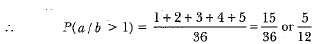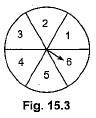Offer running on EduRev: Apply code STAYHOME200 to get INR 200 off on our premium plan EduRev Infinity!

## Mathematics (Maths) Class 10

165 videos|384 docs|115 tests

,

,

,

,

,

,

,

,

,

,

,

,

,

,

,

,

,

,

,

,

,

;